# Region-wise regularization#

In this tutorial we like to demonstrate how to control the regularization of subsurface regions individually by using an ERT field case. The data is a 2d profile that was measured in 2005 on the bottom of a lake. The water body is of course influencing the fields and needs to be treated accordingly.

```
```

We first import pygimli and the modules for ERT and mesh building.

```import pygimli as pg
from pygimli.physics import ert
import pygimli.meshtools as mt
```

## Data and geometry#

The data was measured across a shallow lake with the most electrodes being on the bottom of a lake. We used cables with 2m spaced takeouts.

```data = pg.getExampleData("ert/lake.ohm")
print(data)
```
```Data: Sensors: 48 data: 658, nonzero entries: ['a', 'b', 'err', 'i', 'm', 'n', 'r', 'u', 'valid']
```

The data consists of 658 data with current and voltage using 48 electrodes. We first have a look at the electrode positions measured by a stick.

```pg.plt.plot(pg.x(data), pg.z(data), "o-")
pg.plt.grid();
```On both sides, two electrodes are on shore, but the others are on the bottom of a shallow lake with a maximum depth of 2.5m.

```data["k"] = ert.geometricFactors(data)
data["rhoa"] = data["u"] / data["i"] * data["k"]
ert.show(data);
```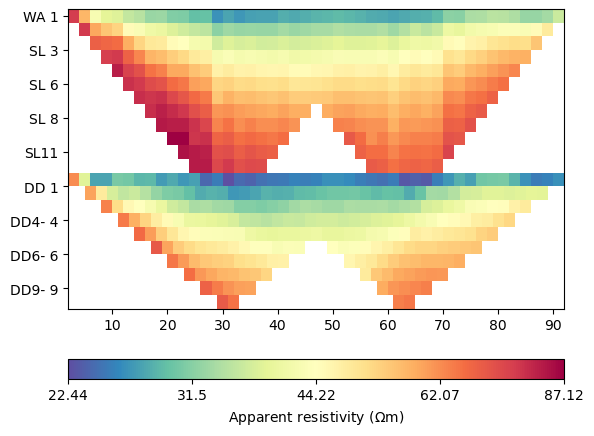```(<matplotlib.axes._subplots.AxesSubplot object at 0x7fe8f5866880>, <matplotlib.colorbar.Colorbar object at 0x7fe8717809d0>)
```

We combined Wenner-Schlumberger (top) and Wenner-beta (bottom) data. The lowest resistivities correspond with the water resistivity of 22.5 \$Omega\$m.

The contained errors are measured standard devitations and should not be used for inversion. Instead, we estimate new errors using 2% and 100microVolts.

```data["err"] = ert.estimateError(data, relativeError=0.02, absoluteUError=1e-4)
print(max(data["err"]))
# pg.show(data, data["err"]*100, label="error (%)");
```
```0.02584795321637427
```

### Building a mesh with the water body#

```# We create a piece-wise linear complex (PLC) as for a case with topography
ax, _ = pg.show(plc, markers=True);
for i, n in enumerate(plc.nodes()[:12]):
ax.text(n.x(), n.y(), str(i))
print(i, n.x(), n.y())
``````0 -4.0 0.0
1 -4.0 -20.0
2 97.7452 -20.0
3 97.7452 0.0
4 -13.37452 0.0
5 -13.37452 -29.37452
6 107.11972 0.0
7 107.11972 -29.37452
8 0.0 0.0
9 1.0 0.0
10 2.0 0.0
11 2.993365 -0.11499999999999999
```

So node number 10 is the left one at the shore

```for i in range(95, plc.nodeCount()):
print(i, plc.node(i).x(), plc.node(i).y())
```
```95 86.7804 -0.5833335
96 87.7715 -0.45
97 88.76255 -0.3166665
98 89.75359999999999 -0.183333
99 90.7494 -0.0916665
100 91.7452 0.0
101 92.7452 0.0
102 93.7452 0.0
```

and 100 the first on the other side. We connect nodes 10 and 100 by an edge

```plc.createEdge(plc.node(10), plc.node(100), marker=-1)
pg.show(plc, markers=True);
```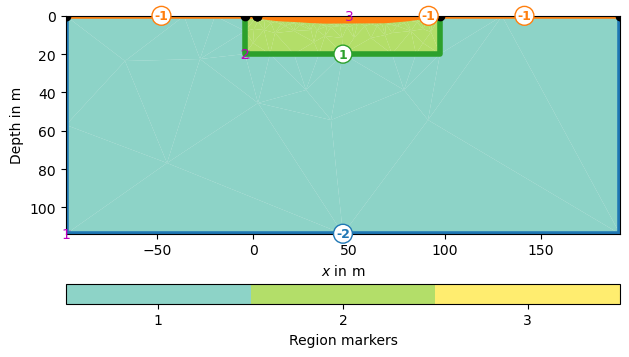```(<matplotlib.axes._subplots.AxesSubplot object at 0x7fe87164b100>, None)
```

As the lake bottom is not a surface boundary (-1) anymore, but an inside boundary, we set its marker >0 by iterating through all boundaries.

```mesh = mt.createMesh(plc, quality=34.4)
for b in mesh.boundaries():
if b.marker() == -1 and not b.outside():
b.setMarker(2)

print(mesh)
pg.show(mesh, markers=True, showMesh=True);
``````Mesh: Nodes: 1136 Cells: 2103 Boundaries: 3238

(<matplotlib.axes._subplots.AxesSubplot object at 0x7fe899f1fbe0>, <matplotlib.colorbar.Colorbar object at 0x7fe8ea3460d0>)
```

## Inversion with the ERT manager#

```mgr = ert.ERTManager(data, verbose=True)
mgr.setMesh(mesh)  # use this mesh for all subsequent runs
mgr.invert()
# mgr.invert(mesh=mesh) would only temporally use the mesh
```
```fop: <pygimli.physics.ert.ertModelling.ERTModelling object at 0x7fe8ab0057c0>
Data transformation: <pygimli.core._pygimli_.RTransLogLU object at 0x7fe899e53d60>
Model transformation: <pygimli.core._pygimli_.RTransLog object at 0x7fe8ab0058b0>
min/max (data): 22.44/87.12
min/max (error): 2%/2.58%
min/max (start model): 47.2/47.2
--------------------------------------------------------------------------------
--------------------------------------------------------------------------------
inv.iter 2 ... chi² = 19.5 (dPhi = 66.94%) lam: 20
--------------------------------------------------------------------------------
inv.iter 3 ... chi² = 7.89 (dPhi = 58.65%) lam: 20.0
--------------------------------------------------------------------------------
inv.iter 4 ... chi² = 5.38 (dPhi = 31.09%) lam: 20.0
--------------------------------------------------------------------------------
inv.iter 5 ... chi² = 3.63 (dPhi = 31.34%) lam: 20.0
--------------------------------------------------------------------------------
inv.iter 6 ... chi² = 2.04 (dPhi = 41.11%) lam: 20.0
--------------------------------------------------------------------------------
inv.iter 7 ... chi² = 2.02 (dPhi = 0.81%) lam: 20.0
################################################################################
#                 Abort criteria reached: dPhi = 0.81 (< 2.0%)                 #
################################################################################

1838 [208.14321162887464,...,96.31630761372877]
```

The fit is obviously not perfect. So we have a look at data and model response.

```ax = mgr.showFit()
```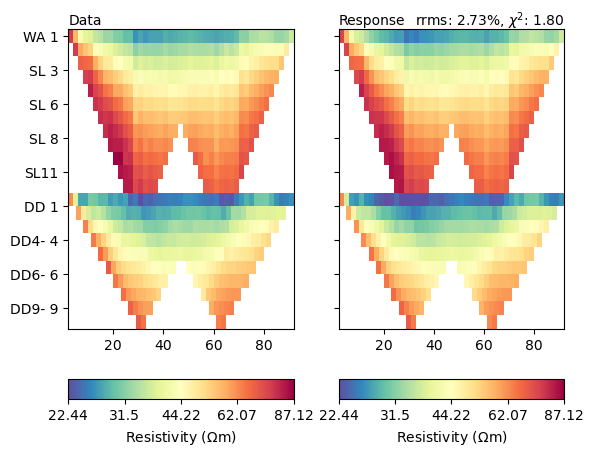Both look very similar, but let us look at the misfit function in detail.

```mgr.showMisfit(errorWeighted=True)
```There is still systematics in the misfit. Ideally it should be a random distribution of Gaussian noise.

```cov = pg.Vector(mgr.model.size(), 1.0)
kw = dict(cMin=20, cMax=300, logScale=True, cMap="Spectral_r", coverage=cov)
ax, cb = mgr.showResult(**kw)
```Apparently, the two regions are already decoupled from each other which makes sense. Let us look in detail at the water cells by extracting the water body.

```water = mesh.createSubMesh(mesh.cells(mesh.cellMarkers() == 3))
resWater = mgr.model[len(mgr.model)-water.cellCount():]
ax, cb = pg.show(water, resWater)
```Apparently, all values are below the expected 22.5\$Omega\$m and some are implausibly low. Therefore we should try to limit them. Moreover, the subsurface structures do not look very “layered”, which is why we make the smoothness anisotropic.

```mgr.inv.setRegularization(zWeight=0.1)
mgr.invert()
# mgr.invert(zWeight=0.1)  # only temporarily
ax, cb = mgr.showResult(**kw)
``````fop: <pygimli.physics.ert.ertModelling.ERTModelling object at 0x7fe8ab0057c0>
Data transformation: <pygimli.core._pygimli_.RTransLogLU object at 0x7fe899e53d60>
Model transformation (cumulative):
0 <pygimli.core._pygimli_.RTransLogLU object at 0x7fe8a90c70a0>
1 <pygimli.core._pygimli_.RTransLogLU object at 0x7fe8a90c70a0>
min/max (data): 22.44/87.12
min/max (error): 2%/2.58%
min/max (start model): 47.2/47.2
--------------------------------------------------------------------------------
--------------------------------------------------------------------------------
inv.iter 2 ... chi² = 12.57 (dPhi = 74.44%) lam: 20
--------------------------------------------------------------------------------
inv.iter 3 ... chi² = 3.19 (dPhi = 74.26%) lam: 20.0
--------------------------------------------------------------------------------
inv.iter 4 ... chi² = 0.97 (dPhi = 68.33%) lam: 20.0

################################################################################
#                  Abort criterion reached: chi² <= 1 (0.97)                   #
################################################################################
```

## Region-specific regularization#

We first want to limit the resistivity of the water between some plausible bounds around our measurements.

```mgr.inv.setRegularization(3, limits=[20, 25], trans="log")
mgr.invert()
ax, cb = mgr.showResult(**kw)
``````fop: <pygimli.physics.ert.ertModelling.ERTModelling object at 0x7fe8ab0057c0>
Data transformation: <pygimli.core._pygimli_.RTransLogLU object at 0x7fe899e53d60>
Model transformation (cumulative):
0 <pygimli.core._pygimli_.RTransLogLU object at 0x7fe8997aee80>
1 <pygimli.core._pygimli_.RTransLogLU object at 0x7fe8997ae4c0>
min/max (data): 22.44/87.12
min/max (error): 2%/2.58%
min/max (start model): 47.2/47.2
--------------------------------------------------------------------------------
--------------------------------------------------------------------------------
inv.iter 2 ... chi² = 7.11 (dPhi = 88.78%) lam: 20
--------------------------------------------------------------------------------
inv.iter 3 ... chi² = 0.99 (dPhi = 84.77%) lam: 20.0

################################################################################
#                  Abort criterion reached: chi² <= 1 (0.99)                   #
################################################################################
```

As a result of the log-log transform, we have a homogeneous body but below the lake bottom values below 20, maybe due to clay content or maybe as compensation of limiting the water resistivity too strong. We could limit the subsurface, too.

```mgr.inv.setRegularization(2, limits=[20, 2000], trans="log")
mgr.invert()
ax, cb = mgr.showResult(**kw)
```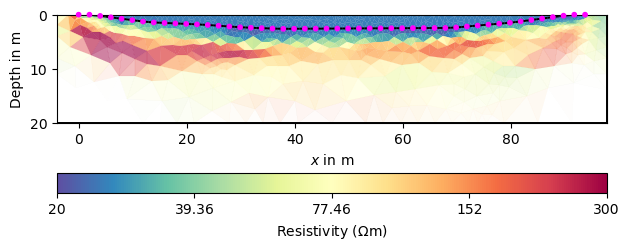```fop: <pygimli.physics.ert.ertModelling.ERTModelling object at 0x7fe8ab0057c0>
Data transformation: <pygimli.core._pygimli_.RTransLogLU object at 0x7fe899e53d60>
Model transformation (cumulative):
0 <pygimli.core._pygimli_.RTransLogLU object at 0x7fe8ab327f40>
1 <pygimli.core._pygimli_.RTransLogLU object at 0x7fe8fa7b0dc0>
min/max (data): 22.44/87.12
min/max (error): 2%/2.58%
min/max (start model): 47.2/47.2
--------------------------------------------------------------------------------
--------------------------------------------------------------------------------
inv.iter 2 ... chi² = 8.3 (dPhi = 67.62%) lam: 20
--------------------------------------------------------------------------------
inv.iter 3 ... chi² = 1.5 (dPhi = 78.96%) lam: 20.0
--------------------------------------------------------------------------------
inv.iter 4 ... chi² = 0.52 (dPhi = 54.1%) lam: 20.0

################################################################################
#                  Abort criterion reached: chi² <= 1 (0.52)                   #
################################################################################
```

Apparently, this makes it harder to fit the data accurately. So maybe an increased clay content can be responsible for resistivity below 20\$Omega\$m in the mud.

## Model reduction#

Another option is to treat the water body as a homogeneous body with only one unknown in the inversion.

```mgr.inv.setRegularization(limits=[0, 0], trans="log")
mgr.inv.setRegularization(3, single=True)
mgr.invert()
ax, cb = mgr.showResult(**kw)

print(mgr.inv.model)
print(min(mgr.model))
```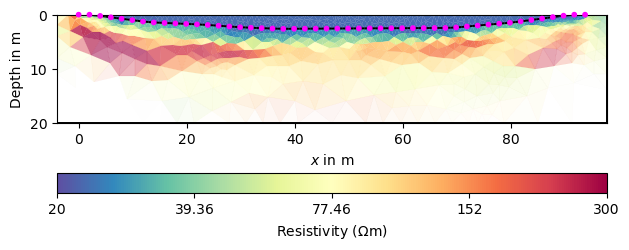```fop: <pygimli.physics.ert.ertModelling.ERTModelling object at 0x7fe8ab0057c0>
Data transformation: <pygimli.core._pygimli_.RTransLogLU object at 0x7fe899e53d60>
Model transformation (cumulative):
0 <pygimli.core._pygimli_.RTransLogLU object at 0x7fe899a7cdc0>
1 <pygimli.core._pygimli_.RTransLogLU object at 0x7fe8ea2f3e80>
min/max (data): 22.44/87.12
min/max (error): 2%/2.58%
min/max (start model): 47.2/47.2
--------------------------------------------------------------------------------
--------------------------------------------------------------------------------
inv.iter 2 ... chi² = 9.95 (dPhi = 62.96%) lam: 20
--------------------------------------------------------------------------------
inv.iter 3 ... chi² = 1.99 (dPhi = 77.96%) lam: 20.0
--------------------------------------------------------------------------------
inv.iter 4 ... chi² = 0.54 (dPhi = 65.24%) lam: 20.0

################################################################################
#                  Abort criterion reached: chi² <= 1 (0.54)                   #
################################################################################
1406 [32.320138266282186,...,21.80517248258542]
21.80517248258542
```

The last value represents the value for the lake, close to our measurement. This value can, however, also be set beforehand.

```mgr.inv.setRegularization(3, fix=22.5)
mgr.invert()
ax, cb = mgr.showResult(**kw)
``````fop: <pygimli.physics.ert.ertModelling.ERTModelling object at 0x7fe8ab0057c0>
Data transformation: <pygimli.core._pygimli_.RTransLogLU object at 0x7fe899e53d60>
Model transformation (cumulative):
0 <pygimli.core._pygimli_.RTransLogLU object at 0x7fe8fa7ef820>
min/max (data): 22.44/87.12
min/max (error): 2%/2.58%
min/max (start model): 47.2/47.2
--------------------------------------------------------------------------------
--------------------------------------------------------------------------------
inv.iter 2 ... chi² = 6.15 (dPhi = 67.51%) lam: 20
--------------------------------------------------------------------------------
inv.iter 3 ... chi² = 1.24 (dPhi = 77.13%) lam: 20.0
--------------------------------------------------------------------------------
inv.iter 4 ... chi² = 0.51 (dPhi = 47.97%) lam: 20.0

################################################################################
#                  Abort criterion reached: chi² <= 1 (0.51)                   #
################################################################################
```

We see that the lake does not appear anymore as it is not a part of the inversion mesh `mgr.paraDomain` anymore.

Instead of the standard smoothness we use geostatistical regularization.

```mgr.inv.setRegularization(2, correlationLengths=[30, 2])
mgr.invert()
ax, cb = mgr.showResult(**kw)
``````fop: <pygimli.physics.ert.ertModelling.ERTModelling object at 0x7fe8ab0057c0>
Data transformation: <pygimli.core._pygimli_.RTransLogLU object at 0x7fe899e53d60>
Model transformation (cumulative):
0 <pygimli.core._pygimli_.RTransLogLU object at 0x7fe8fa7b0dc0>
min/max (data): 22.44/87.12
min/max (error): 2%/2.58%
min/max (start model): 47.2/47.2
--------------------------------------------------------------------------------
--------------------------------------------------------------------------------
inv.iter 2 ... chi² = 3.06 (dPhi = 76.35%) lam: 20
--------------------------------------------------------------------------------
inv.iter 3 ... chi² = 0.61 (dPhi = 76.06%) lam: 20.0

################################################################################
#                  Abort criterion reached: chi² <= 1 (0.61)                   #
################################################################################
```

## Region coupling#

In case (does not make sense here) the two regions should be coupled to each other, you can set so-called inter-region constraints.

```mgr = ert.ERTManager(data, verbose=True)
mgr.setMesh(mesh)
print(mgr.fop.regionManager().regionCount())
mgr.inv.setRegularization(cType=1, zWeight=0.2)
mgr.fop.setInterRegionCoupling(2, 3, 1.0)  # normal coupling
mgr.invert()
ax, cb = mgr.showResult(**kw)
```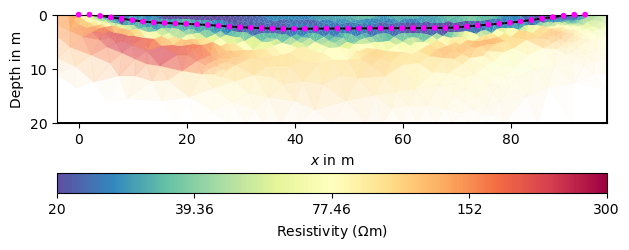```3
fop: <pygimli.physics.ert.ertModelling.ERTModelling object at 0x7fe8999df3b0>
Data transformation: <pygimli.core._pygimli_.RTransLogLU object at 0x7fe8999df630>
Model transformation (cumulative):
0 <pygimli.core._pygimli_.RTransLogLU object at 0x7fe8f54d81c0>
1 <pygimli.core._pygimli_.RTransLogLU object at 0x7fe8aae826a0>
min/max (data): 22.44/87.12
min/max (error): 2%/2.58%
min/max (start model): 47.2/47.2
--------------------------------------------------------------------------------
--------------------------------------------------------------------------------
inv.iter 2 ... chi² = 10.43 (dPhi = 67.94%) lam: 20
--------------------------------------------------------------------------------
inv.iter 3 ... chi² = 1.95 (dPhi = 80.02%) lam: 20.0
--------------------------------------------------------------------------------
inv.iter 4 ... chi² = 0.55 (dPhi = 65.97%) lam: 20.0

################################################################################
#                  Abort criterion reached: chi² <= 1 (0.55)                   #
################################################################################
```

The general image is of course similar, but the structures are mirrored around the lake bottom. Moreover the resistivity in the lake is far too high. Note that all of the obtained images are equivalent with respect to data and errors.

### Take-away messages#

• always have a look at the data fit and get hands on data errors

• a lot of different models are able to fit the data, particularly in the full space

• regions can be very specifically controlled

• constrain or fix whenever possibly (and reliable)

• sometimes geostatistic constraints outperform classical smoothness but sometimes not

• play with regularization and keep looking at data fit

Total running time of the script: ( 1 minutes 52.318 seconds)

Gallery generated by Sphinx-Gallery